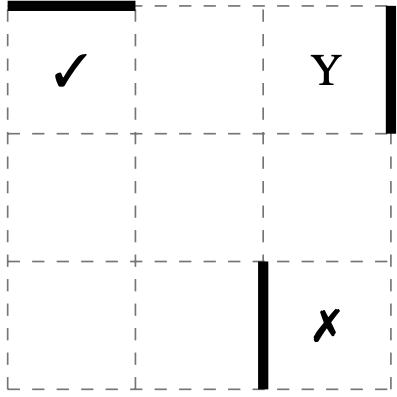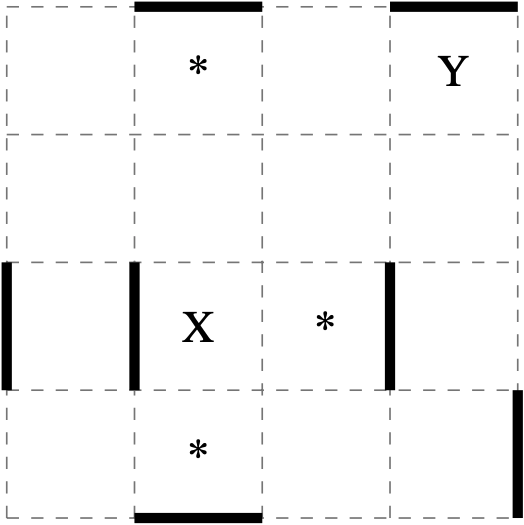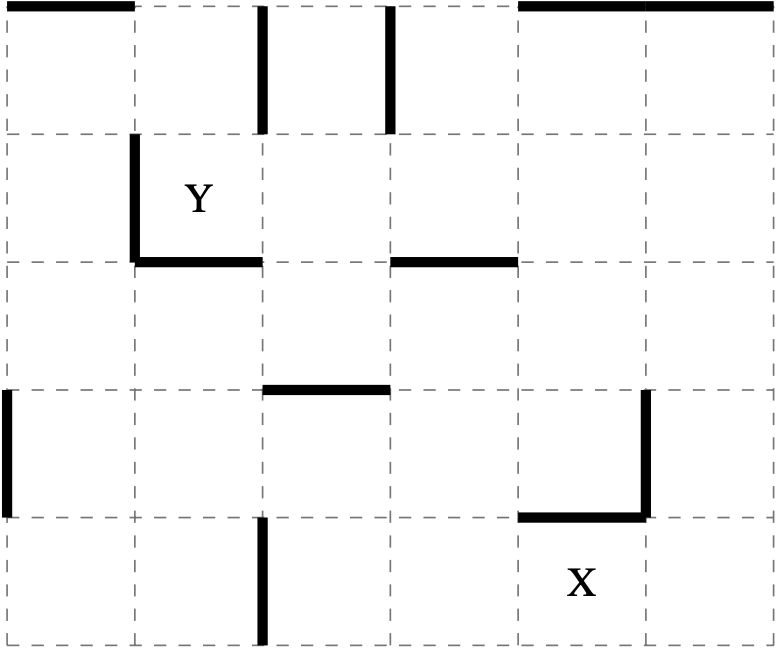Astrid the astronaut is floating in a grid. Each time she pushes off she keeps gliding until she collides with a solid wall, marked by a thicker line.

From such a wall she can propel herself either parallel or perpendicular to the wall, but always travelling directly $$\leftarrow, \rightarrow, \uparrow, \downarrow$$. Floating out of the grid means death.

In grid below, Astrid can reach square Y from square . But if she starts from square there is no wall to stop her and she will float past Y and out of the grid.In following grid, from square $$\text{X}$$ Astrid can float to three different squares with one push (each is marked with *).

Push $$\leftarrow$$ is not possible from $$\text{X}$$ due to the solid wall to the left.

From $$\text{X}$$ it takes three pushes to stop safely at square $$\text{Y}$$, namely $$\downarrow, \rightarrow, \uparrow$$.

The sequence $$\uparrow, \rightarrow$$ would have Astrid float past $$\text{Y}$$ and out of the grid.In the grid below, what is the least number of pushes that Astrid can make to safely travel from $$\text{X}$$ to $$\text{Y}$$?